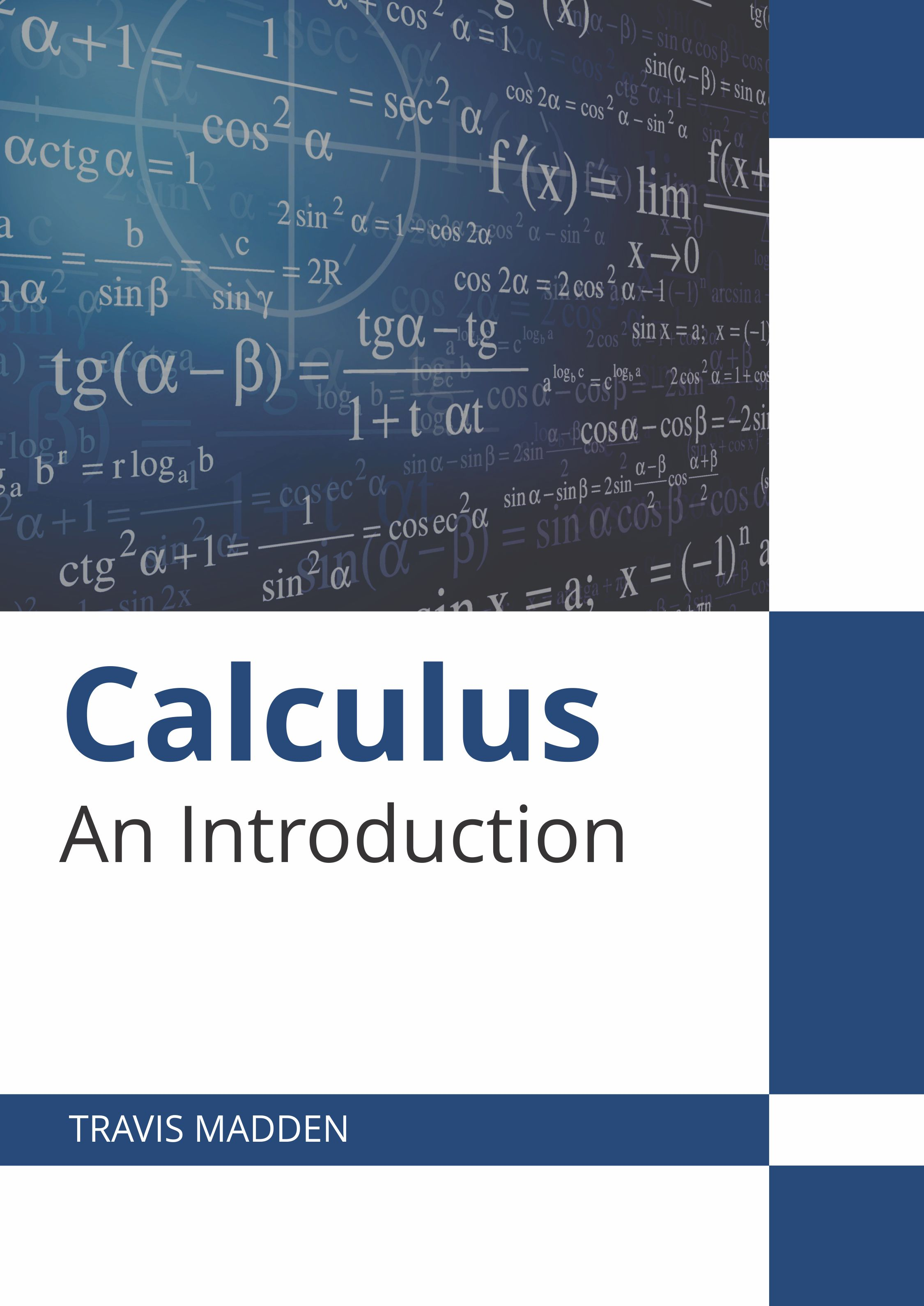BROWSE BY

#### Author

Results 1 - 2 of 2Calculus: An Introduction Author : Travis Madden Subject : Mathematics Calculus refers to the mathematical study of continuous change. The major branches of calculus are differential calculus and integral calculus. Differential calculus is concerned with the immediate rate Read MoreCalculus: An Introduction Author : Travis Madden Subject : Mathematics Calculus refers to the mathematical study of continuous change. The major branches of calculus are differential calculus and integral calculus. Differential calculus is concerned with the immediate rate Read More
Results 1 - 2 of 2